# NCERT Solutions For Class 6 Maths Fractions Exercise 7.5

ncert textbook

## NCERT Solutions For Class 6 Maths Fractions Exercise 7.5

NCERT Solutions for Class 6 Maths Chapter 7 Fractions Ex 7.5

Exercise 7.5

Question 1.
Write these fractions appropriately as additions or subtractions.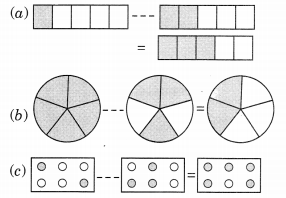Solution:
(a) The given figure represents the addition ofThus the given diagrams can be represented as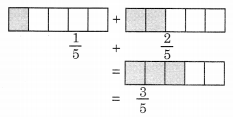(b) The given figure represents the difference between 1 and$\frac { 3 }{ 5 }$.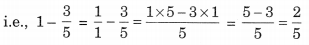Thus, the given diagrams can be represented as(c) The given figure represents addition of$\frac { 2 }{ 6 }$and$\frac { 3 }{ 6 }$.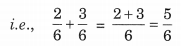Thus, the given diagrams can be represented as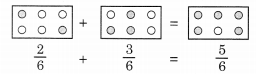Question 2.
Solve:Solution:Question 3.
Shubham painted$\frac { 2 }{ 3 }$of the wall space in his room. His sister Madhavi helped and painted$\frac { 1 }{ 3 }$of the wall space. How much did they paint together?
Solution:
Fraction of wall painted by Shubham =$\frac { 2 }{ 3 }$
Fraction of wall painted by Madhavi =$\frac { 1 }{ 3 }$
Fraction of wall painted by Shubham and MadhaviThus the fraction of wall painted by both = 1

Question 4.
Fill in the missing fractions.Solution: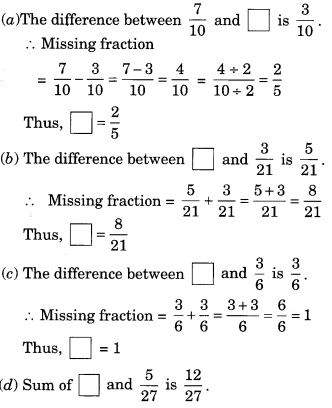Question 5.
Javed was given$\frac { 5 }{ 7 }$of a basket of oranges. What fraction of oranges was left in the basket?
Solution:
Fraction of basket of oranges =$\frac { 5 }{ 7 }$
Fraction of basket as a whole can be taken as 1.
∴ Fraction of basket of oranges leftThus, the required fraction =$\frac { 2 }{ 7 }$.